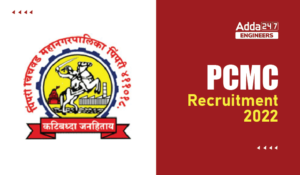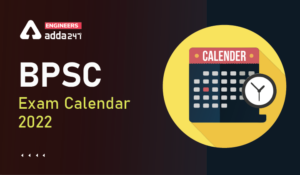Engineering Jobs   »   QUIZ : CIVIL ENGINEER ( 29-Sep-2020)

# QUIZ : CIVIL ENGINEER ( 29-Sep-2020)

Quiz: Civil Engineering
Exam: UPPSC-AE
Topic: Miscellaneous

Each question carries 1 mark
Negative marking: 1/3 mark
Time: 10 Minutes

Q1. In Casagrande’s liquid limit device, the material of the test specimen is harder than the standard rubber. This hardness indicates that the liquid limit, plasticity index, flow index and toughness index, respectively, of the specimen, are
(a) more, less more and same
(b) same, less, same and more
(c) less, less, same and less
(d) less, same, less and more

Q2. What is the minimum value of test results (in N/mm²) for compressive strength compliance requirement for concrete M 20 as per codal provision?
(a) f_ck-1
(b) f_ck-5
(c) f_ck-3
(d) f_ck-4

Q3. Which one of the following is categorized as a long term loss of prestress in a prestressed concrete member
(a) Loss due to elastic shortening
(b) Loss due to friction
(c) Loss due to relaxation of strands-
(d) Loss due to anchorage slip.

Q4. If L_f is load factor S is shape factor and F is factor of safety in elastic design, the following:
(a) L_f=S×F
(b) L_f=S+F
(c) L_f=S-F
(d) L_f=F-S

Q5. Group symbols assigned to silty sand and clayey sand are respectively
(a) SS and CS
(b) SM and CS
(c) SM and SC
(d) MS and CS

Q6. The reduction coefficient of a reinforced concrete column with an effective length of 4.5 m and size 250×300 mm² us _______:
(a) 0.8
(b) 0.85
(c) 0.9
(d) 0.95

Q7. Both Reynolds and Froude numbers assume significance in one of the example.
(a) Motion of submarine at large depths.
(b) Motion of ship in deep seas
(c) crushing of a missile in Air.
(d) flow over spill way

Q8. If fineness modulus of a sand is 2.5, it is graded as
(a) coarse sand
(b) medium sand
(c) very fine sand
(d) fine sand

Q9. In a slab, the minimum reinforcement using steel of grade Fe 415 is –
(a) 0.12% of is gross sectional area
(b) 0.20% of is gross sectional area
(c) 0.15% of is gross sectional area
(d) 0.10% of is gross sectional area

Q10. If the maximum bending moment of a simply supported slab is M kg – cm, the effective depth of the slab is
(a) M/100Q
(b) M/(10√Q)
(c) √(M/Q)
(d) √(M/(100 Q))

SOLUTION
S1. Ans.(c)
Sol. If the test specimen is harder than the standard rubber less number of blows will fill the groove for a given water content. In other words 25 number of blows will be required at less water content. So liquid limit and thereby plasticity index will be less. There will be no effect on flow index (I_f) Toughness index = I_p/I_f will also be less.
S2. Ans.(d)
Sol. As per IS : 456 – 2000, the characteristic compressive strength compliance requirement are-
Grade of Concrete Individual test results (in N/mm²)
M 15 ≥ f_ck – 3
M 20 or above ≥ f_ck – 4
f_ck = Characteristic strength of concrete.

S3. Ans.(c)

S4. Ans.(a)
Sol. Load factor = Shape factor × factor of safety
▭(L_F=S×F)
▭(Shape factor (S)=Z_P/Z_e )
Where Z_P = plastic section modulus
Z_e = Elastic section modulus
S5. Ans.(c)

S6. Ans.(c)
Sol. reduction coefficient (Cr) = 1.25 – l_eff/(48.b)
Cr=1.25-4500/(48×250)
▭(Cr=0.875≈0.9)

S7. Ans.(b)
Sol.

S8. Ans.(d)
Sol.
S.No. Type of sand Fineness modulus
1. Fine sand 2.2 – 2.6
2. Medium sand 2.6 – 2.9
3. Coarse sand 2.9 – 3.2
S9. Ans.(a)
Sol. Minimum reinforcement.
For mild steel bar → 0.15% of gross area
For HYSD bar → 0.12% of gross area

S10. Ans.(d)
Sol. moment of resistance (M) = Qd² (N-mm)
M/(10×10) (kg-cm)=Qd^2
▭(d=√(M/100Q))

Sharing is caring!

Thank You, Your details have been submitted we will get back to you.
•MP Vyapam Sub Engineer Notification 2022...
•PCMC Recruitment 2022, Check here the de...
•BEL Recruitment 2022, Check here For 13 ...
•Indian Air Force Apprentice Recruitment ...
•BPSC Exam Calendar 2022, Check BPSC Assi...
•SSC Scientific Assistant IMD Recruitment...